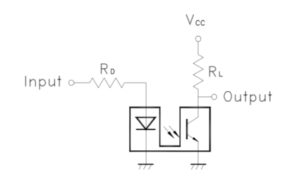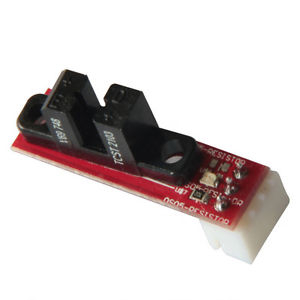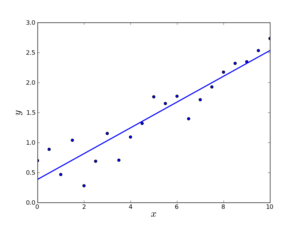# Robot Car – p4: Adding Speed Control

We have seen in that the prototype has a issue with keeping a straight direction. The magnetometer is not reacting fast enough for controlling the motor speed precisely.

## Optocoupler

The solution to is problem is adding coded wheels. The coded wheels where delivered with the car motor and wheel set but without the necessary electronic. A coded wheel is just a small disc with radial slots. The sensor you need to add is a led coupled to a photo transistor. You can buy an optocoupler like the LTH 301-07 but is that case you’ll have to solder two resistance to get the right current to the LED and the phototransistor.If you are not willing to do some soldering a simple solution is to buy a end stop like the one used in 3D printers.## Counting Impulses

The optocoupler will send impulses with an interval depending of the wheel rotating speed. If you want to measure precisely the speed of the wheel you need either to count the time between two impulses or how many impulses you get in a time span. For doing that the easiest solution I found was to map each optocoupler to an interrupt of the arduino. When the signal raises we just trigger the interrupt and we add 1 to a counter.

```// left Motor
const int leftSpeedInt = 0;
const int leftMotorCtrl1 = 2;
const int leftMotorCtrl2 = 3;
const int leftMotorEnable = 10;

// right Motor
const int rightSpeedInt = 1;
const int rightMotorCtrl1 = 4;
const int rightMotorCtrl2 = 5;
const int rightMotorEnable = 11;

Wheel leftWheel = Wheel(expander,leftMotorCtrl1,leftMotorCtrl2,leftMotorEnable);
Wheel rightWheel = Wheel(expander,rightMotorCtrl1,rightMotorCtrl2,rightMotorEnable);

void leftWheelInt() {
leftWheel.incSpeedCount();
}
void rightWheelInt() {
rightWheel.incSpeedCount();
}
void setup() {
attachInterrupt(leftSpeedInt,leftWheelInt,RISING);
attachInterrupt(rightSpeedInt,rightWheelInt,RISING);
}```

In this code I use a class to encapsulate all motor control features.

## Smoothing Data:

The loop runs around every 70ms this gives for the number of impulses between two loops around 10. This number is not very high and a difference of  “1” changes the calculated speed if you divide the number of impulses by the time between 2 loops. I decided to use a linear regression to calculate the speed.

```#include <LinearRegression.h>

Fifo::Fifo() {
_first=0;
_last=0;
_size=0;
}

void Fifo::push(double value) {
_buff[_last]=value;
_last=(_last+1)%queueLength;
_size++;
int i;
}

double Fifo::pop() {
double value= _buff[_first];
_first=(_first+1)%queueLength;
_size--;
return value;
}

int Fifo::getSize() {
return _size;
}

LinearRegression::LinearRegression() {
n=0;
sum_X=0.0;
sum_Y=0.0;
sum_X2=0.0;
sum_XY=0.0;
}```
```void LinearRegression::add(double X,double Y) {
n+=1;
sum_X+=double(X);
sum_Y+=double(Y);
sum_X2+=double(X)*double(X);
sum_XY+=double(X)*double(Y);
}

void LinearRegression::sub(double X,double Y) {
n-=1;
sum_X-=double(X);
sum_Y-=double(Y);
sum_X2-=double(X)*double(X);
sum_XY-=double(X)*double(Y);
}

double LinearRegression::getCoefficient() {
if (n==1)
return sum_Y/sum_X;
return (sum_XY - (sum_X*sum_Y)/n) / ( sum_X2 - sum_X*sum_X/n);
}

double LinearRegression::getIntercept() {
return predict(0.0);
}```
```double LinearRegression::predict(double X) {
double coef = getCoefficient();
return double(coef*(X-sum_X/n) + sum_Y/n);
}

RunningLinearRegression::RunningLinearRegression(int nbPoints) {
list_X=Fifo();
list_Y=Fifo();
_nbPoints=nbPoints;
_linearRegression = LinearRegression();

};

if (list_X.getSize() == _nbPoints ) {
_linearRegression.sub(list_X.pop(),list_Y.pop());
}
list_X.push(X);
list_Y.push(Y);
}

double RunningLinearRegression::getCoefficient() {
return _linearRegression.getCoefficient();
}

double RunningLinearRegression::getIntercept() {
return _linearRegression.getIntercept();
}

double RunningLinearRegression::predict(double X) {
return _linearRegression.predict(X);
}```

Is this code you can see that we can compute the average speed as the coefficient of the regression line between. In our case we keep the last ten point to calculate the speed. If we consider that each point is distant of 70ms the last 10 points span over 700ms. Less than a second seems reasonable considering the acceleration that our car is capable of.## Speed control with PID:

Now that we are able to compute the rotation speed with enough precision, we hit another problem: setting the “enable” input of the H bridge to get the expected speed. The motors are controlled by a H-bridge. Each motor need 3 pins to be controlled:

• 2 pins to control the rotation direction: 0-0 stopped, 1-0 frontward, 0-1 backward
• 1 pin to set the “speed”

In fact in that case what we control is just the amount of current sent to the motor which is correlated to the speed but not enough to predict the speed. In fact the actual rotation speed depends of the motor, the surface on which we are rolling, the level of charge of the battery,… To make sure that we get the expected speed we need to feedback the measured speed to the controler setting the level of current sent to the motor. This controller is called PID for proportional–integral–derivative controllerThe idea is to apply a linear combination of the value, its derivative and its integral to find the proper output level. For more detail I recommend to read the wikipedia definition. The good news is that you can find some Arduino libraries implementing PID.

After all these efforts for controlling the motor speed I was please to see that my car was now running in a straight line when setting the same speed for both wheels on the PID controller. This maybe looks to you as a small achievement but this is a necessary step if you want to control the direction of the car.

In the next post we we’ll get the car assembled and start testing it.

Code can be found at: https://github.com/gvtech/arduino/tree/master/robot_car_PID